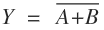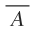# NOR Gate – Symbol, Truth table & Circuit

A NOR gate is a digital logic gate that implements logical NOR operation. It is a combination of an OR gate and NOT gate. If we connect the output of OR gate to the input of a NOT gate, the gate so obtained is known as NOR gate. This gate is also called as Negated OR gate. It is a universal gate.

Contents

In Boolean expression, the NOR gate is expressed asand is being read as “A or B negated” or “A or B bar”.

## NOR  gate Symbol

The logic symbol of NOR gate is shown in figure 1(a).

Figure 1(b) shows the NOR gate as the combination of OR gate and NOT gate.

## NOR gate truth table

The truth table for figure 1(a) is shown below.

 A B0 0 1 0 1 0 1 0 0 1 1 0

The truth table for figure 1(b) is shown below.

 A B X = A+B0 0 0 1 0 1 1 0 1 0 1 0 1 1 1 0

From the above truth table, it can be concluded that the output will be logical 1 or high when all inputs are at logical 0 or low.

## NOR gate as Universal gate

A universal gate is a gate which can implement any Boolean function without the need to use any other gate types. The NAND and NOR gates are universal gates.

The repeated use of the NOR gate can produce all other logic gates. Thus in digital circuits, it serves as a building block.

### NOT gate using NOR gate

To produce NOT gate using NOR gate, the two inputs are joined together as shown in figure 2.

The truth table of the above combination is given below.

 Input (A) Output (Y =) 0 1 1 0

### AND gate using NOR gate

To produce AND gate using NOR gate, the two output of the NOT gates (made from NOR gate by joining the two inputs) are connected to the NOR gate as shown in fig. 3.

The truth table of the above combination is given below.

 A B X Z Y = A.B 0 0 1 1 0 0 1 1 0 0 1 0 0 1 0 1 1 0 0 1

### OR gate using NOR gate

To produce OR gate using NOR gate, the output of the NOR gate is fed as input to NOT gate (made from NOR gate by joining the two inputs) as shown in figure 4.

The truth table of the above combination is given below.

 A B X Y = A+B 0 0 1 0 0 1 0 1 1 0 0 1 1 1 0 1

Q1. What is the speciality of NOR gate?

Answer. The speciality of this gate is that it is a universal gate and can perform all the basic logical operation.

Q2. What is the use of De Morgan’s theorem?

Answer. De Morgan’s theorem is extremely useful in simplification of boolean expressions in which a sum or product of variables is inverted.

Q3. What is the difference between canonical form and standard form?

Answer. In canonical form, a boolean function is expressed as sum of minterms or product of maxterms and is obtained by reading a function from the truth table. Canonical forms may or may not contain the least numbers of literals because, by definition, each max term or minterm must contain all variables, complemented or uncomplemented.

In standard form, the terms of function may contain one, two or any number of literals. Standard form is expressed as sum of products or product of sums.

Q4. The complete set of only those logic gates designated as universal gates is

1. NOT, OR and AND gates
2. XNOR, NOR and NAND gates
3. NOR and NAND gates
4. XNOR, OR and NAND gates